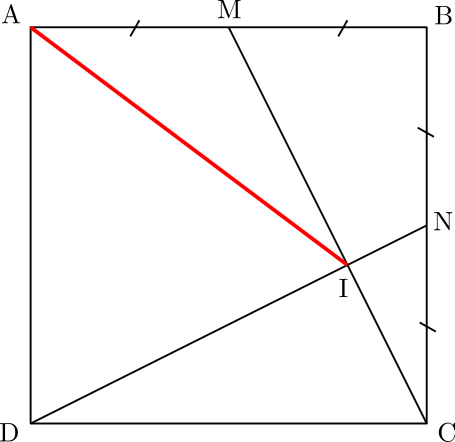# Squares and midpoints

Geometry Level 3Let $ABCD$ be a square with side length 1, $M$ and $N$ be the midpoint of line segments $AB$ and $BC$ respectively, and $CM$ and $DN$ cut at point $I$. What is the length of $AI$?

×